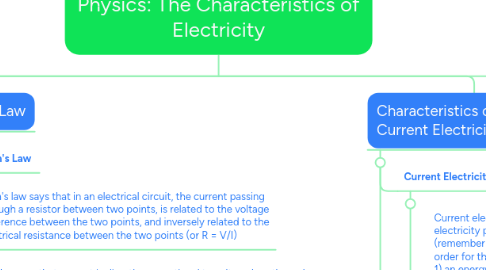# Physics: The Characteristics of ElectricityEthan Gallant
Get Started. It's FreePhysics: The Characteristics of Electricity## 1. Characteristics of Electricity: Static Electricity

### 1.3. Electrostatics is the study of static electricity.

1.3.1. Creating Static Electricity

1.3.2. There are 3 main ways to build up stationary charges (static electricity) on objects. These are by friction, contact (touch) and induction.

1.3.3. Friction is the force resisting the relative motion of two surfaces in contact with each other. The rubbing of the surface of one object on that of another causes this friction. The force of friction can remove electrons from one object and cause them to transfer to the other object. As one object loses electrons, the other object gains them. Then, because they are oppositely charged, they attract each other.

1.3.4. Electrons can also be transferred through contact or just by touching a charged object to a neutral object. In this case, the neutral object gains the same type of charge as the object that touched it. The end result is two similarly charged objects.

1.3.5. Induction is the movement of electrons within a substance, caused by a nearby charged object, without direct contact between the substance and the object. The induced charge is USUALLY a temporary charge and is only detected as long as the charged object comes close to the neutral one.

1.3.5.1. Remember

1.3.5.2. Only electrons move from one object to another, protons do not move!

1.3.5.3. Opposite charges attract.

1.3.5.4. Similar charges repel.

1.3.5.4.1. Questions

1.3.5.4.2. What is static electricity? What are the 3 ways we can generate static electricity? Describe each in detail.

1.3.5.4.3. Study the triboelectric series.

1.3.5.4.4. What is the difference between materials that are conductors and insulators? Give examples.

## 2. Characteristics of Electricity: Current Electricity

### 2.1. Current Electricity

2.1.1. Current electricity is the electricity produced by the continuous flow of electrons (remember only electrons move, protons do not). In order for this flow to happen we require two things: 1) an energy source – such as a battery or electrochemical cell 2) a complete path – created by connecting wires

### 2.2. Potential Difference, Current, and Resistance

2.2.1. In a battery, the electrons gain stored energy, called potential energy. By connecting a wire to the battery, the electrons can then leave by the negative electrode and move through the circuit until it returns to the battery by the positive electrode. Throughout the circuit, the potential energy the electron started with has been used for motion, or has been converted to other forms of energy by a load. Therefore, at different points of the circuit, there is a difference in energy which we call the potential difference or voltage. The symbol for voltage is V.

2.2.2. Electric current is a measure of the amount of electric charge that passes a point in a circuit each second. The symbol for current is I.

2.2.3. Resistance is the degree to which a substance opposes the flow of electric current through it. All substances resist electron flow to some extent. The symbol for resistance is R.

2.2.3.1. Circuit Diagrams

2.2.3.2. The four main parts of an electric circuit are the load (what the electricity is powering) [e.g. light bulbs, heaters, e.t.c...]), the switch (what switches the power on and off by closing and opening the path), the conducting wire (what allows the flow of electrons), and the electrical source (what creates the power source [e.g. battery]).

2.2.3.3. There are two different types of circuits: series and parallel. Series circuits are one path only, allowing electrons to only flow in one direction. This means if anywhere on the path is cut off, the whole circuits power is cut off. Parallel circuits have multiple paths, so cutting off certain parts of the path will usually have no affect on power flow.

2.2.3.3.1. Questions

2.2.3.3.2. List and describe the functions of 4 components of an electric circuit.

2.2.3.3.3. What is potential difference? Why would electrons leaving a resistor have less potential energy than when they entered?

2.2.3.3.4. What is current? How does increased resistance in a wire affect electrical current?

2.2.3.3.5. Draw a circuit diagram of a SERIES circuit with a battery, connecting wires, one light bulb, and two resistors.

2.2.3.3.6. Draw a circuit diagram of a PARALLEL circuit with a battery, connecting wires, one light bulb, and two resistors.

2.2.3.3.7. Explain why the flow of current is different in series and parallel circuits.

2.2.3.3.8. Explain why the voltage/potential difference used by loads is different series and parallel circuits.

## 4. Ohm's Law

### 4.4. This can be written in three ways: I = V/R, V = I*R, and R = V/I.

4.4.1. Questions

4.4.2. A current of 1.5A flows through a 30Ω resistor that is connected across a battery. Find the voltage of the battery.

4.4.3. A 180V outlet has an appliance that draws a current of 10A connected to it. What is the resistance of the appliance?

4.4.4. A 12Ω light bulb is in a series circuit powered by a 6V battery. What is the current of the circuit?

4.4.5. In a circuit where voltage is kept constant, what happens to current if resistance is doubled?